# Sorting Algorithms

Posted by Leonardo Galler and Matteo Kimura on April 21, 2019

# What are Sorting Algorithms?

Sorting algorithms are ways to organize an array of items from smallest to largest. These algorithms can be used to organize messy data and make it easier to use. Furthermore, having an understanding of these algorithms and how they work is fundamental for a strong understanding of Computer Science which is becoming more and more critical in a world of premade packages. This blog focuses on the speed, uses, advantages, and disadvantages of specific Sorting Algorithms.

Although there is a wide variety of sorting algorithms, this blog explains Straight Insertion, Shell Sort, Bubble Sort, Quick Sort, Selection Sort, and Heap Sort. The first two algorithms (Straight Insertion and Shell Sort) sort arrays with insertion, which is when elements get inserted into the right place. The next 2 (Bubble Sort and Quick Sort) sort arrays with exchanging which is when elements move around the array. The last one is heap sort which sorts through selection where the right elements are selected as the algorithm runs down the array.

## Big-O Notation

Before this blog goes any further, it is essential to explain the methods that professionals use to analyze and assess algorithm complexity and performance. The current standard is called “Big O notation” named according to its notation which is an “O” followed by a function such as “O(n).”

### Formal definition

Suppose f(x) and g(x) are two functions defined on some subset of the real numbers. We write f(x) = O(g(x)) (or f(x) = O(g(x)) for x -> ∞ to be more precise) if and only if there exist constants N and C such that |f(x)| <= C |g(x)| for all x>N. Intuitively, this means that f does not grow faster than g.

Big O is used to denote either the time complexity of an algorithm or how much space it takes up. This blog focuses mainly on the time complexity part of this notation. The way people can calculate this is by identifying the worst case for the targeted algorithm and formulating a function of its performance given an n amount of elements. For example, if there were an algorithm that searched for the number 2 in an array, then the worst case would be if the 2 was at the very end of the array. Therefore, the Big O notation would be O(n) since it would have to run through the entire n-element array before finding the number 2.

To help you, find below a table with algorithms and its complexity.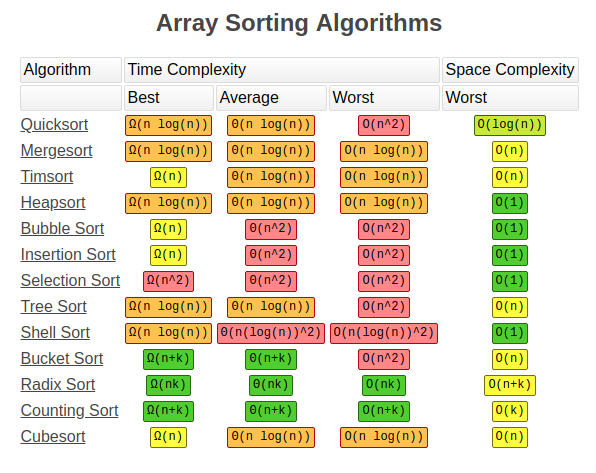## Straight Insertion SortStraight insertion sort is one of the most basic sorting algorithms that essentially inserts an element into the right position of an already sorted list. It is usually added at the end of a new array and moves down until it finds an element smaller thank itself (the desired position). The process repeats for all the elements in the unsorted array. Consider the array {3,1,2,5,4}, we begin at 3, and since there are no other elements in the sorted array, the sorted array becomes just {3}. Afterward, we insert 1 which is smaller than 3, so it would move in front of 3 making the array {1,3}. This same process is repeated down the line until we get the array {1,2,3,4,5}.The advantages of this process are that it is straightforward and easy to implement. Also, it is relatively quick when there are small amounts of elements to sort. It can also turn into binary insertion which is when you compare over longer distances and narrow it down to the right spot instead of comparing against every single element before the right place. However, a straight insertion sort is usually slow whenever the list becomes large.

Main Characteristics:

• Insertion sort family
• Straightforward and simple
• Worst case = O(n^2)

Python implementation:

#!/usr/bin/env python3
# -*- coding: utf-8 -*-
"""
Created on Sun Mar 10 17:13:56 2019

@note: Insertion sort algorithm
@source: http://interactivepython.org/courselib/static/pythonds/SortSearch/TheInsertionSort.html

"""

def insertionSort(alist):
for index in range(1,len(alist)):

currentvalue = alist[index]
position = index

while position>0 and alist[position-1]>currentvalue:
alist[position]=alist[position-1]
position = position-1

alist[position]=currentvalue


## Shell Sort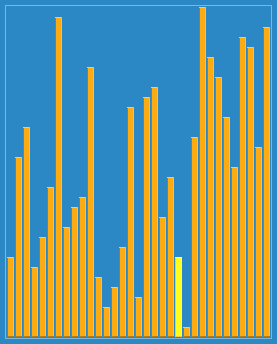Shell sort is an insertion sort that first partially sorts its data and then finishes the sort by running an insertion sort algorithm on the entire array. It generally starts by choosing small subsets of the array and sorting those arrays. Afterward, it repeats the same process with larger subsets until it reaches a point where the subset is the array, and the entire thing becomes sorted. The advantage of doing this is that having the array almost entirely sorted helps the final insertion sort achieve or be close to its most efficient scenario.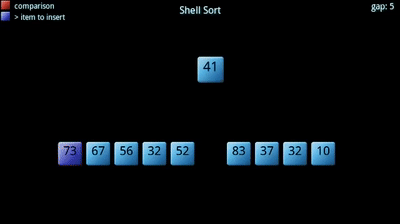Furthermore, increasing the size of the subsets is achieved through a decreasing increment term. The increment term essentially chooses every kth element to put into the subset. It starts large, leading to smaller (more spread out) groups, and it becomes smaller until it becomes 1 (all of the array).

The main advantage of this sorting algorithm is that it is more efficient than a regular insertion sort. Also, there is a variety of different algorithms that seek to optimize shell sort by changing the way the increment decreases since the only restriction is that the last term in the sequence of increments is 1. The most popular is usually Knuth’s method which uses the formula h=((3^k)-1)/2 giving us a sequence of intervals of 1 (k=1),4 (k=2),13 (k=3), and so on. On the other hand, shell sort is not as efficient as other sorting algorithms such as quicksort and merge sort.

Main Characteristics:

• Sorting by insertion
• Can optimize algorithm by changing increments
• Using Knuth’s method, the worst case is O(n^(3/2))

Python implementation:

#!/usr/bin/env python3
# -*- coding: utf-8 -*-
"""
Created on Sun Mar 10 17:13:56 2019

@note: Insertion sort - Shell Method algorithm
@source: https://www.w3resource.com/python-exercises/data-structures-and-algorithms/python-search-and-sorting-exercise-7.php

"""

def shellSort(alist):
sublistcount = len(alist)//2
while sublistcount > 0:
for start_position in range(sublistcount):
gap_InsertionSort(alist, start_position, sublistcount)

sublistcount = sublistcount // 2

def gap_InsertionSort(nlist,start,gap):
for i in range(start+gap,len(nlist),gap):

current_value = nlist[i]
position = i

while position>=gap and nlist[position-gap]>current_value:
nlist[position]=nlist[position-gap]
position = position-gap

nlist[position]=current_value


## Bubble Sort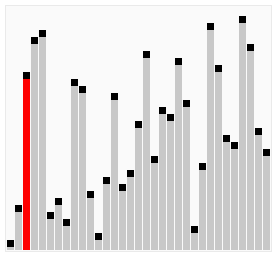Bubble sort compares adjacent elements of an array and organizes those elements. Its name comes from the fact that large numbers tend to “float” (bubble) to the top. It loops through an array and sees if the number at one position is greater than the number in the following position which would result in the number moving up. This cycle repeats until the algorithm has gone through the array without having to change the order. This method is advantageous because it is simple and works very well for mostly sorted lists. As a result, programmers can quickly and easily implement this sorting algorithm. However, the tradeoff is that this is one of the slower sorting algorithms.

Main Characteristics: Exchange sorting Easy to implement Worst Case = O(n^2)

Python implementation:

#!/usr/bin/env python3
# -*- coding: utf-8 -*-
"""
Created on Sun Mar 10 18:05:51 2019

@note: Exchanging sort - Bubble Sort algorithm
@source: http://interactivepython.org/runestone/static/pythonds/SortSearch/TheBubbleSort.html

"""

def bubbleSort(alist):
for passnum in range(len(alist)-1,0,-1):
for i in range(passnum):
if alist[i]>alist[i+1]:
temp = alist[i]
alist[i] = alist[i+1]
alist[i+1] = temp


## QuicksortQuicksort is one of the most efficient sorting algorithms, and this makes of it one of the most used as well. The first thing to do is to select a pivot number, this number will separate the data, on its left are the numbers smaller than it and the greater numbers on the right. With this, we got the whole sequence partitioned. After the data is partitioned, we can assure that the partitions are oriented, we know that we have bigger values on the right and smaller values on the left. The quicksort uses this divide and conquer algorithm with recursion. So, now that we have the data divided we use recursion to call the same method and pass the left half of the data, and after the right half to keep separating and ordinating the data. At the end of the execution, we will have the data all sorted.

Main characteristics:

• From the family of Exchange Sort Algorithms
• Worst case complexity O(n²)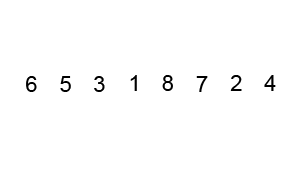Python implementation:

#!/usr/bin/env python3
# -*- coding: utf-8 -*-
"""
Created on Sun Mar 10 18:09:35 2019

@note: Exchanging sort - Bubble Sort algorithm
@source: http://interactivepython.org/courselib/static/pythonds/SortSearch/TheQuickSort.html

"""

def quickSort(alist):
quickSortHelper(alist,0,len(alist)-1)

def quickSortHelper(alist,first,last):
if first<last:

splitpoint = partition(alist,first,last)

quickSortHelper(alist,first,splitpoint-1)
quickSortHelper(alist,splitpoint+1,last)

def partition(alist,first,last):
pivotvalue = alist[first]

leftmark = first+1
rightmark = last

done = False
while not done:

while leftmark <= rightmark and alist[leftmark] <= pivotvalue:
leftmark = leftmark + 1

while alist[rightmark] >= pivotvalue and rightmark >= leftmark:
rightmark = rightmark -1

if rightmark < leftmark:
done = True
else:
temp = alist[leftmark]
alist[leftmark] = alist[rightmark]
alist[rightmark] = temp

temp = alist[first]
alist[first] = alist[rightmark]
alist[rightmark] = temp

return rightmark


## Heapsort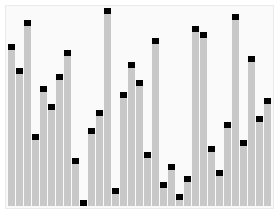Heapsort is a sorting algorithm based in the structure of a heap. The heap is a specialized data structure found in a tree or a vector. In the first stage of the algorithm, a tree is created with the values to be sorted, starting from the left, we create the root node, with the first value. Now we create a left child node and insert the next value, at this moment we evaluate if the value set to the child node is bigger than the value at the root node, if yes, we change the values. We do this to all the tree. The initial idea is that the parent nodes always have bigger values than the child nodes.

At the end of the first step, we create a vector starting with the root value and walking from left to right filling the vector.

Now we start to compare parent and child nodes values looking for the biggest value between them, and when we find it, we change places reordering the values. In the first step, we compare the root node with the last leaf in the tree. If the root node is bigger, then we change the values and continue to repeat the process until the last leaf is the larger value. When there are no more values to rearrange, we add the last leaf to the vector and restart the process. We can see this in the image below.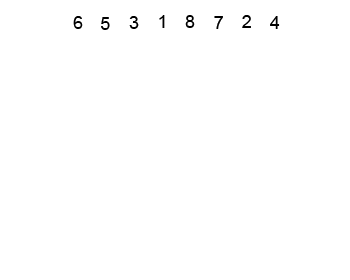The main characteristics of the algorithm are:

• From the family of sorting by selection
• Comparisons in the worst case = O(n log n)
• Not stable

Python implementation:

#!/usr/bin/env python3
# -*- coding: utf-8 -*-
"""
Created on Sun Mar 10 18:18:25 2019

@source: https://www.geeksforgeeks.org/heap-sort/

"""
# Python program for implementation of heap Sort

# To heapify subtree rooted at index i.
# n is size of heap
def heapify(arr, n, i):
largest = i # Initialize largest as root
l = 2 * i + 1	 # left = 2*i + 1
r = 2 * i + 2	 # right = 2*i + 2

# See if left child of root exists and is
# greater than root
if l < n and arr[i] < arr[l]:
largest = l

# See if right child of root exists and is
# greater than root
if r < n and arr[largest] < arr[r]:
largest = r

# Change root, if needed
if largest != i:
arr[i],arr[largest] = arr[largest],arr[i] # swap

# Heapify the root.
heapify(arr, n, largest)

# The main function to sort an array of given size
def heapSort(arr):
n = len(arr)

# Build a maxheap.
for i in range(n, -1, -1):
heapify(arr, n, i)

# One by one extract elements
for i in range(n-1, 0, -1):
arr[i], arr = arr, arr[i] # swap
heapify(arr, i, 0)

heapSort(arr)



## Benchmark: How fast they are?

After development of the algorithms it is good for us to test how fast they can be. In this part we developed a simple program using the code above to generate a basic benchmark, just to see how much time they can use to sort a list of integers. Important observations about the code:

• Python default recursion call limit is 1,000, in this test we are using big numbers, so we needed to improve that number to run the benchmark without errors. The limit was set to 10,000.
• This code just measure the running time of each algorithm.
• It was made 20 tests with different size of lists ranging from 2500 to 50000.
• The numbers were generate randonly ranging from 1 to 10000. The results are the following: Shell sort and Heap Sort algorithms performed well despite the length of the lists, in the other side we found that Insertion sort and Bubble sort algorithms were far the worse, increasing largely the computing time. See the results in the chart below.## Conclusion

In this post, we showed 5 of the most common sorting algorithms used today. Before using any of them is extremely important to know how fast it runs and how much space is going to use. So it’s the tradeoff between complexity, speed, and volume. Another critical characteristic of the sorting algorithms that are important to know is its stability. The stability means that the algorithm keeps the order of elements with equal key values. The best algorithm changes for each different set of data and as a result, understanding our data plays a significant role in the process of choosing the right algorithm.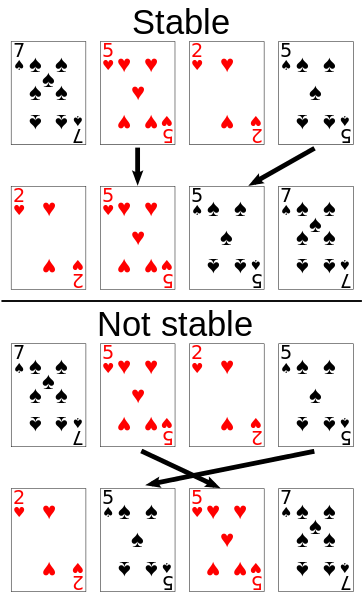As we can see, understanding our data plays a very important role in the process of choosing the right algorithm.

If this post got your attention, take a look at video below, it will give you a concise explanation about 15 sorting algorithms.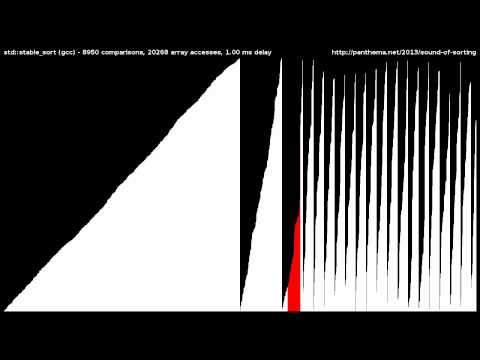References:

Big O Notation - https://en.wikipedia.org/wiki/Big_O_notation

Knuth, Donald Ervin, 1938 - The art of computer programming / Donald Ervin Knuth. xiv,782 p. 24 cm.

15 Sorting Algorithms in 6 minutes - https://www.youtube.com/watch?v=kPRA0W1kECg

Insertion sort - https://pt.wikipedia.org/wiki/Insertion_sort

Insertion sort - http://interactivepython.org/courselib/static/pythonds/SortSearch/TheInsertionSort.html

Shell sort - https://en.wikipedia.org/wiki/Shellsort

Shell sort - http://www.bebetterdeveloper.com/algorithms/sorting/sorting-shell-sort.html

Shell sort - https://www.researchgate.net/publication/234791427_ENHANCED_SHELL_SORT_ALGORITHM

Shell sort - https://www.w3resource.com/python-exercises/data-structures-and-algorithms/python-search-and-sorting-exercise-7.php

Bubble sort - https://en.wikibooks.org/wiki/A-level_Computing/AQA/Paper_1/Fundamentals_of_algorithms/Sorting_algorithms#Bubble_Sort

Quicksort - https://commons.wikimedia.org/wiki/File:Quicksort.gif

Quicksort - http://interactivepython.org/courselib/static/pythonds/SortSearch/TheQuickSort.html

Heapsort Algorithm - https://en.wikipedia.org/wiki/Heapsort

Heapsort Algorithm - https://www.geeksforgeeks.org/heap-sort/

Bubble sort - http://interactivepython.org/runestone/static/pythonds/SortSearch/TheBubbleSort.html